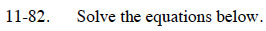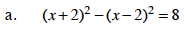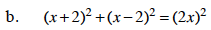Home > CCA2 > Chapter 11 > Lesson 11.2.4 > Problem11-82

11-82.
1. Solve the equations below. Homework Help ✎

1. (x + 2)2 − (x − 2)2 = 8

2. (x + 2)2 + (x − 2)2 = (2x)2Expand the binomials before distributing −1.

x = 1Square each term and simplify.